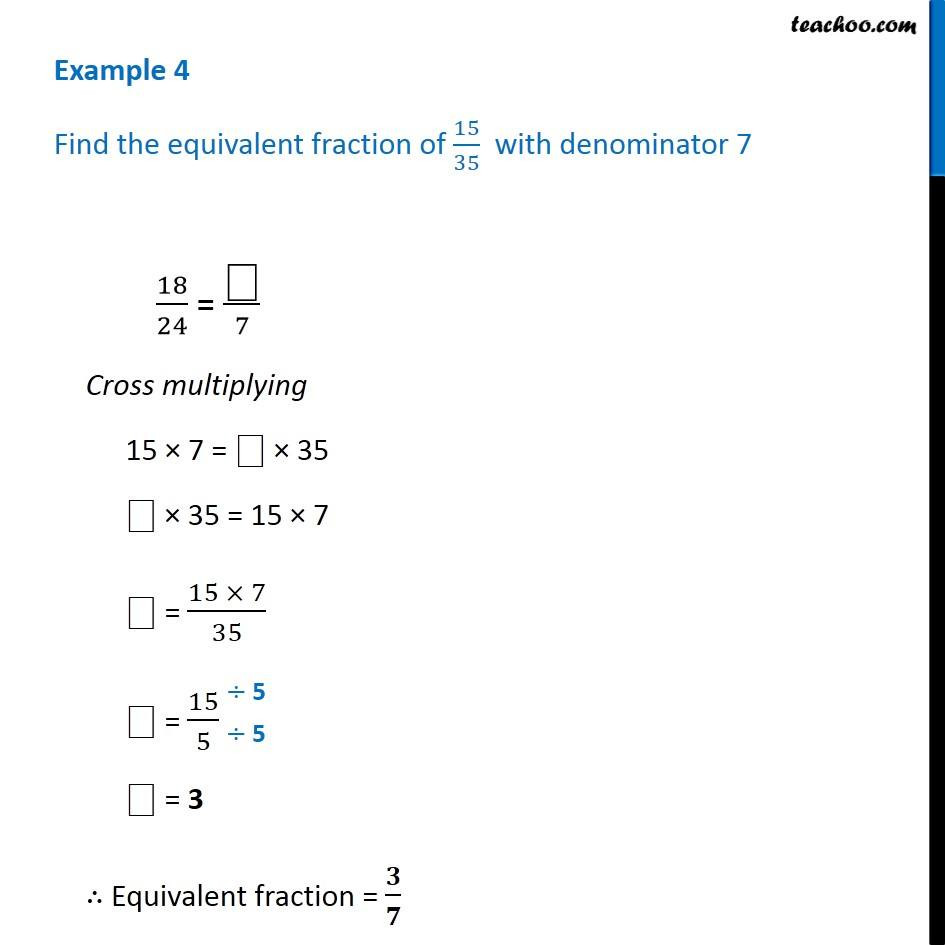Examples

Chapter 7 Class 6 Fractions
Serial order wiseLearn in your speed, with individual attention - Teachoo Maths 1-on-1 Class

### Transcript

Example 4 Find the equivalent fraction of 15/35 with denominator 7 18/24 = " " /7 Cross multiplying 15 7 = " " 35 " " 35 = 15 7 " " = (15 7)/35 " " = 15/5 " " = 3 Equivalent fraction = /